# Snub cube

Snub cube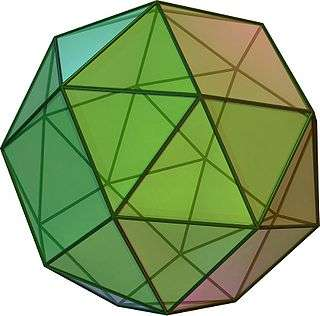TypeArchimedean solid
Uniform polyhedron
ElementsF = 38, E = 60, V = 24 (χ = 2)
Faces by sides(8+24){3}+6{4}
Conway notationsC
Schläfli symbolssr{4,3} orht0,1,2{4,3}
Wythoff symbol| 2 3 4
Coxeter diagram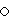Symmetry groupO, 1/2B3, [4,3]+, (432), order 24
Rotation groupO, [4,3]+, (432), order 24
Dihedral Angle3-3: 153°14′04″ (153.23°)
3-4: 142°59′00″ (142.98°)
ReferencesU12, C24, W17
PropertiesSemiregular convex chiral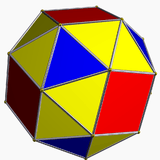Colored faces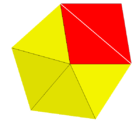3.3.3.3.4
(Vertex figure)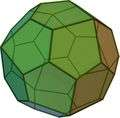Pentagonal icositetrahedron
(dual polyhedron)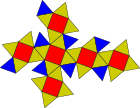Net

In geometry, the snub cube, or snub cuboctahedron, is an Archimedean solid with 38 faces: 6 squares and 32 equilateral triangles. It has 60 edges and 24 vertices.

It is a chiral polyhedron, that is, it has two distinct forms, which are mirror images (or "enantiomorphs") of each other. The union of both forms is a compound of two snub cubes, and the convex hull of both sets of vertices is a truncated cuboctahedron.

Kepler first named it in Latin as cubus simus in 1619 in his Harmonices Mundi. H. S. M. Coxeter, noting it could be derived equally from the octahedron as the cube, called it snub cuboctahedron, with a vertical extended Schläfli symbol.

## Dimensions

For a snub cube with edge length 1, its surface area is 6 + 83 and its volume iswhere t is the tribonacci constantIf the original snub cube has edge length 1, its dual pentagonal icositetrahedron has side lengths.

## Cartesian coordinates

Cartesian coordinates for the vertices of a snub cube are all the even permutations of

(±1, ±1/t, ±t)

with an even number of plus signs, along with all the odd permutations with an odd number of plus signs, where t  1.83929 is the tribonacci constant. Taking the even permutations with an odd number of plus signs, and the odd permutations with an even number of plus signs, gives a different snub cube, the mirror image. Taking all of them together yields the compound of two snub cubes.

This snub cube has edges of length α, a number which satisfies the equationand can be written asTo get a snub cube with unit edge length, divide all the coordinates above by the value α given above.

## Orthogonal projections

The snub cube has two special orthogonal projections, centered, on two types of faces: triangles, and squares, correspond to the A2 and B2 Coxeter planes.

Orthogonal projections
Centered by Face
Triangle
Face
Square
Edge
Image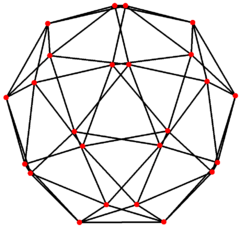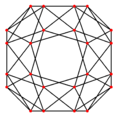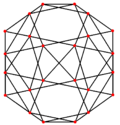Projective
symmetry
 + 
Dual
image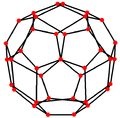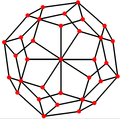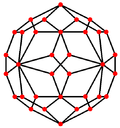## Spherical tiling

The snub cube can also be represented as a spherical tiling, and projected onto the plane via a stereographic projection. This projection is conformal, preserving angles but not areas or lengths. Great circle arcs (geodesics) on the sphere are projected as circular arcs on the plane.

Orthographic projection Stereographic projection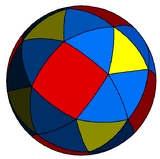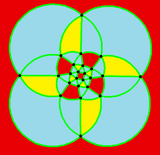square-centered

## Geometric relations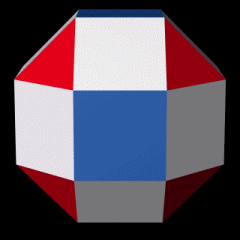A snub cube can be constructed from a transformed rhombicuboctahedron by rotating the 6 blue square faces until the 12 white squares become pairs of equilateral triangles.

The snub cube can be generated by taking the six faces of the cube, pulling them outward so they no longer touch, then giving them each a small rotation on their centers (all clockwise or all counter-clockwise) until the spaces between can be filled with equilateral triangles.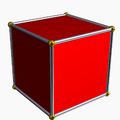Cube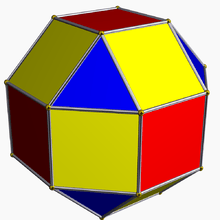Rhombicuboctahedron(Expanded cube)Snub cube

It can also be constructed as an alternation of a nonuniform omnitruncated cube, deleting every other vertex and creating new triangles at the deleted vertices. A properly proportioned (nonuniform) great rhombicuboctahedron will create equilateral triangles at the deleted vertices. Depending on which set of vertices are alternated, the resulting snub cube can have a clockwise or counterclockwise twist.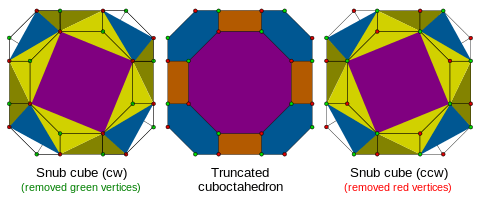An "improved" snub cube, with a slightly smaller square face and slightly larger triangular faces compared to Archimedes' uniform snub cube, is useful as a spherical design.

The snub cube is one of a family of uniform polyhedra related to the cube and regular octahedron.

This semiregular polyhedron is a member of a sequence of snubbed polyhedra and tilings with vertex figure (3.3.3.3.n) and Coxeter–Dynkin diagram. These figures and their duals have (n32) rotational symmetry, being in the Euclidean plane for n = 6, and hyperbolic plane for any higher n. The series can be considered to begin with n=2, with one set of faces degenerated into digons.

The snub cube is second in a series of snub polyhedra and tilings with vertex figure 3.3.4.3.n.

## Snub cubical graph

Snub cubical graph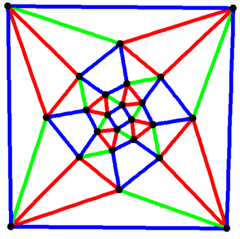4-fold symmetry
Vertices 24
Edges 60
Automorphisms 24
Properties Hamiltonian, regular

In the mathematical field of graph theory, a snub cubical graph is the graph of vertices and edges of the snub cube, one of the Archimedean solids. It has 24 vertices and 60 edges, and is an Archimedean graph.